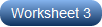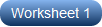How about evaluating functions that are in the form f(x) = ax2 + bx + c? Reaffirm the fact that quadratic functions have the highest degree 2, and their graph is a parabola. Instruct students to put the like terms together and bring the function rule to the standard form. Substituting the value of x and solving for f(x) is what students are expected to do. The easy level involves positive and negative integers, while the moderate level incorporates both decimals and fractions. Dealing with quadratic functions is no more a tough nut to crack with the ample practice provided by these worksheets. Practice adding, subtracting, multiplying, and dividing to evaluate the quadratic functions in these printable worksheets. Give our free worksheet a try!

Get high school students to assign the integer values of x in the quadratic functions that are in the form ax2 + bx + c and solve for f(x) in these evaluating quadratic functions worksheets.Test the skills of high school students as they upgrade to substituting decimal and fractional values of x in the quadratic functions and solve for f(x) in a variety of exercises.Adequate practice in substituting x-values in quadratic functions is what high school learners will gain as they work their way through these pdfs on evaluating quadratic functions at different values of x.Open the doors to enormous practice with these worksheets on evaluating quadratic functions. Upskill learners as they plug in decimal and fractional values of x in the function and solve.